Documentation

## Wideband Beamforming

### Support for Wideband Beamforming

Beamforming achieved by multiplying the sensor input by a complex exponential with the appropriate phase shift only applies for narrowband signals. In the case of wideband, or broadband, signals, the steering vector is not a function of a single frequency. Wideband processing is commonly used in microphone and acoustic applications.

Phased Array System Toolbox™ software provides conventional and adaptive wideband beamformers. They include:

See Acoustic Beamforming Using a Microphone Array for an example of using wideband beamforming to extract speech signals in noise.

### Time-Delay Beamforming of Microphone ULA Array

This example shows how to perform wideband conventional time-delay beamforming with a microphone array of omnidirectional elements. Create an acoustic (pressure wave) chirp signal. The chirp signal has a bandwidth of 1 kHz and propagates at a speed of 340 m/s at ground level.

Note: This example runs only in R2016b or later. If you are using an earlier release, replace each call to the function with the equivalent `step` syntax. For example, replace `myObject(x)` with `step(myObject,x)`.

```c = 340; t = linspace(0,1,50e3)'; sig = chirp(t,0,1,1000);```

Collect the acoustic chirp with a ten-element ULA. Use omnidirectional microphone elements spaced less than one-half the wavelength at the 50 kHz sampling frequency. The chirp is incident on the ULA with an angle of $6{0}^{\circ }$ azimuth and ${0}^{\circ }$ elevation. Add random noise to the signal.

```microphone = phased.OmnidirectionalMicrophoneElement(... 'FrequencyRange',[20 20e3]); array = phased.ULA('Element',microphone,'NumElements',10,... 'ElementSpacing',0.01); collector = phased.WidebandCollector('Sensor',array,'SampleRate',5e4,... 'PropagationSpeed',c,'ModulatedInput',false); sigang = [60;0]; rsig = collector(sig,sigang); rsig = rsig + 0.1*randn(size(rsig));```

Apply a wideband conventional time-delay beamformer to improve the SNR of the received signal.

```beamformer = phased.TimeDelayBeamformer('SensorArray',array,... 'SampleRate',5e4,'PropagationSpeed',c,'Direction',sigang); y = beamformer(rsig); subplot(2,1,1) plot(t(1:5000),real(rsig(1:5e3,5))) axis([0,t(5000),-0.5,1]) title('Signal (real part) at the 5th element of the ULA') subplot(2,1,2) plot(t(1:5000),real(y(1:5e3))) axis([0,t(5000),-0.5,1]) title('Signal (real part) with time-delay beamforming') xlabel('Seconds')```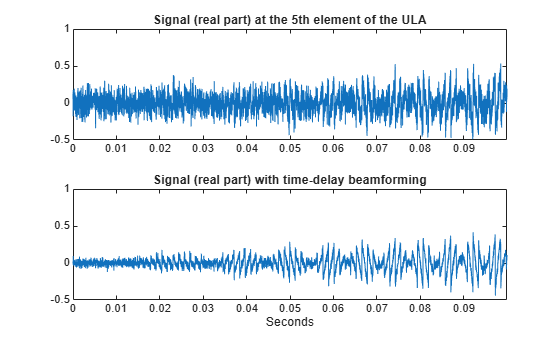### Visualization of Wideband Beamformer Performance

This example shows how to plot the response of an acoustic microphone element and an array of microphone elements to validate the performance of a beamformer. The array must maintain an acceptable array pattern throughout the bandwidth.

Note: This example runs only in R2016b or later. If you are using an earlier release, replace each call to the function with the equivalent `step` syntax. For example, replace `myObject(x)` with `step(myObject,x)`.

Create an 11-element uniform linear array (ULA) of microphones using cosine antenna elements as microphones. The `phased.CosineAntennaElement` System object™ is general enough to be used as a microphone element as well because it creates or receives a scalar field. You need to change the response frequencies to the audible range. In addition make sure the `PropagationSpeed` parameter in the array `pattern` methods are set to the speed of sound in air.

```c = 340; freq = [1000 2750]; fc = 2000; numels = 11; microphone = phased.CosineAntennaElement('FrequencyRange',freq); array = phased.ULA('NumElements',numels,... 'ElementSpacing',0.5*c/fc,'Element',microphone);```

Plot the response pattern of the microphone element over a set of frequencies.

```plotFreq = linspace(min(freq),max(freq),15); pattern(microphone,plotFreq,[-180:180],0,'CoordinateSystem','rectangular',... 'PlotStyle','waterfall','Type','powerdb')```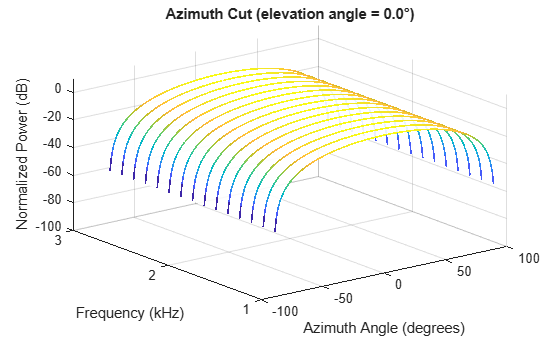This plot shows that the element pattern is constant over the entire bandwidth.

Plot the response pattern of an 11-element array over the same set of frequencies.

```pattern(array,plotFreq,[-180:180],0,'CoordinateSystem','rectangular',... 'PlotStyle','waterfall','Type','powerdb','PropagationSpeed',c)```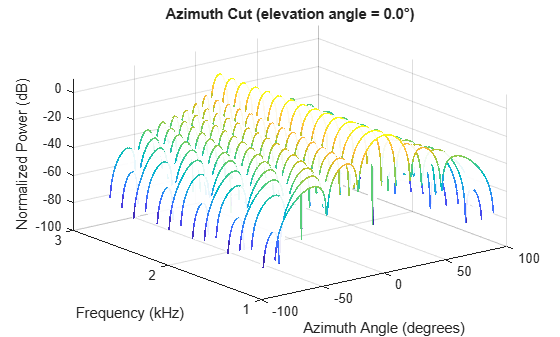This plot shows that the element pattern mainlobe decreases with frequency.

Apply a subband phase shift beamformer to the array. The direction of interest is 30° azimuth and 0° elevation. There are 8 subbands.

```direction = [30;0]; numbands = 8; beamformer = phased.SubbandPhaseShiftBeamformer('SensorArray',array,... 'Direction',direction,... 'OperatingFrequency',fc,'PropagationSpeed',c,... 'SampleRate',1e3,... 'WeightsOutputPort',true,'SubbandsOutputPort',true,... 'NumSubbands',numbands); rx = ones(numbands,numels); [y,w,centerfreqs] = beamformer(rx);```

Plot the response pattern of the array using the weights and center frequencies from the beamformer.

```pattern(array,centerfreqs.',[-180:180],0,'Weights',w,'CoordinateSystem','rectangular',... 'PlotStyle','waterfall','Type','powerdb','PropagationSpeed',c)```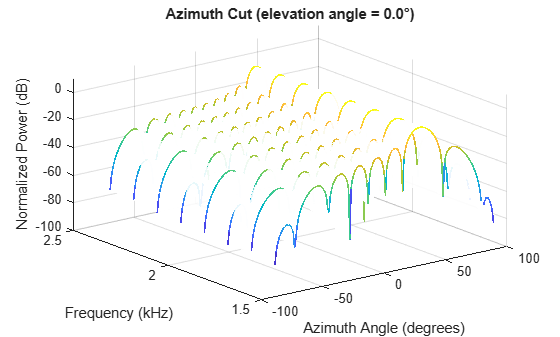The above plot shows the beamformed pattern at the center frequency of each subband.

Plot the response pattern at three frequencies in two-dimensions.

```centerfreqs = fftshift(centerfreqs); w = fftshift(w,2); idx = [1,5,8]; pattern(array,centerfreqs(idx).',[-180:180],0,'Weights',w(:,idx),'CoordinateSystem','rectangular',... 'PlotStyle','overlay','Type','powerdb','PropagationSpeed',c) legend('Location','South')```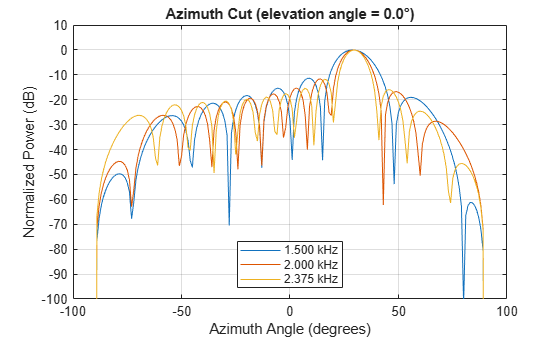This plot shows that the main beam direction remains constant while the beamwidth decreases with frequency.